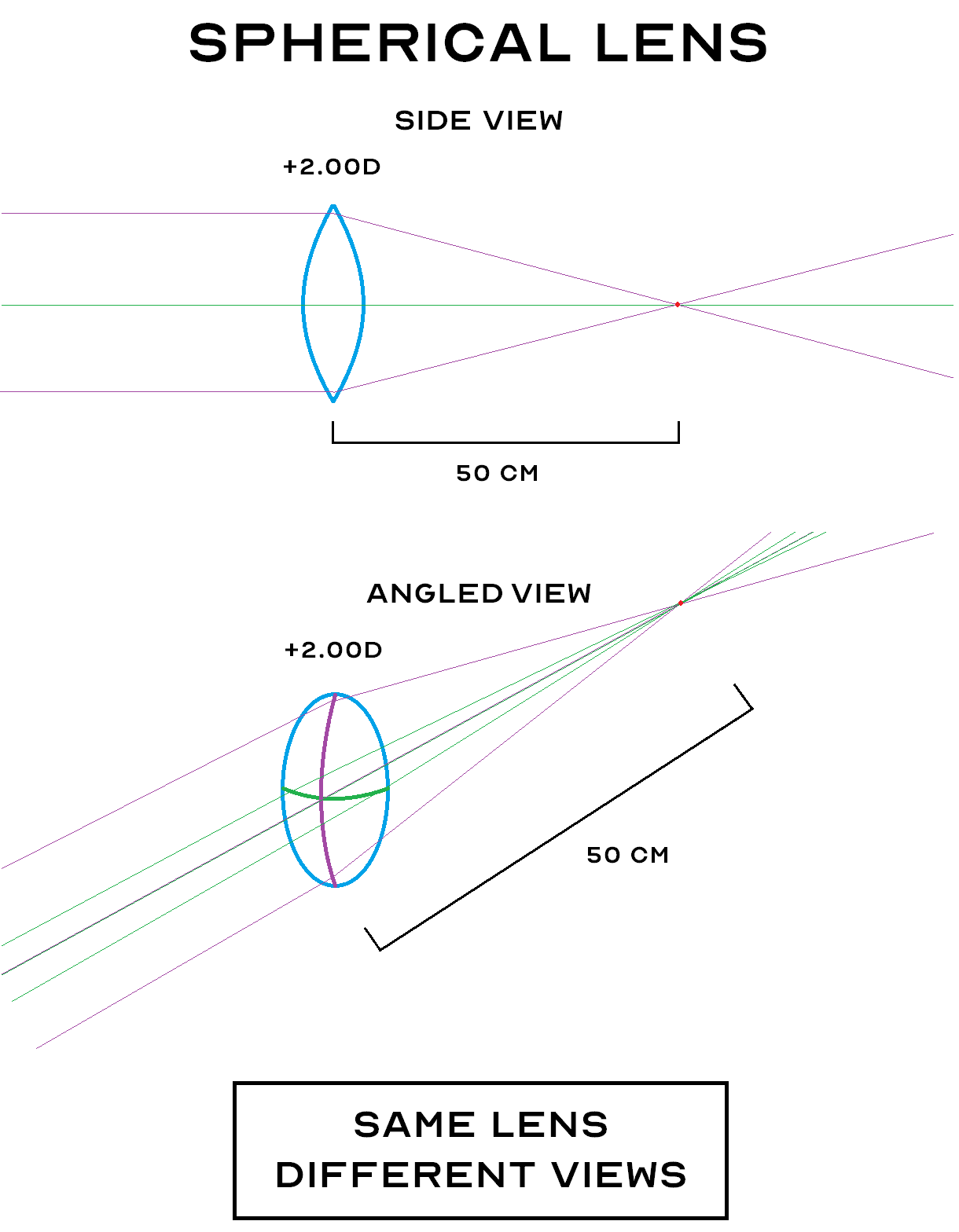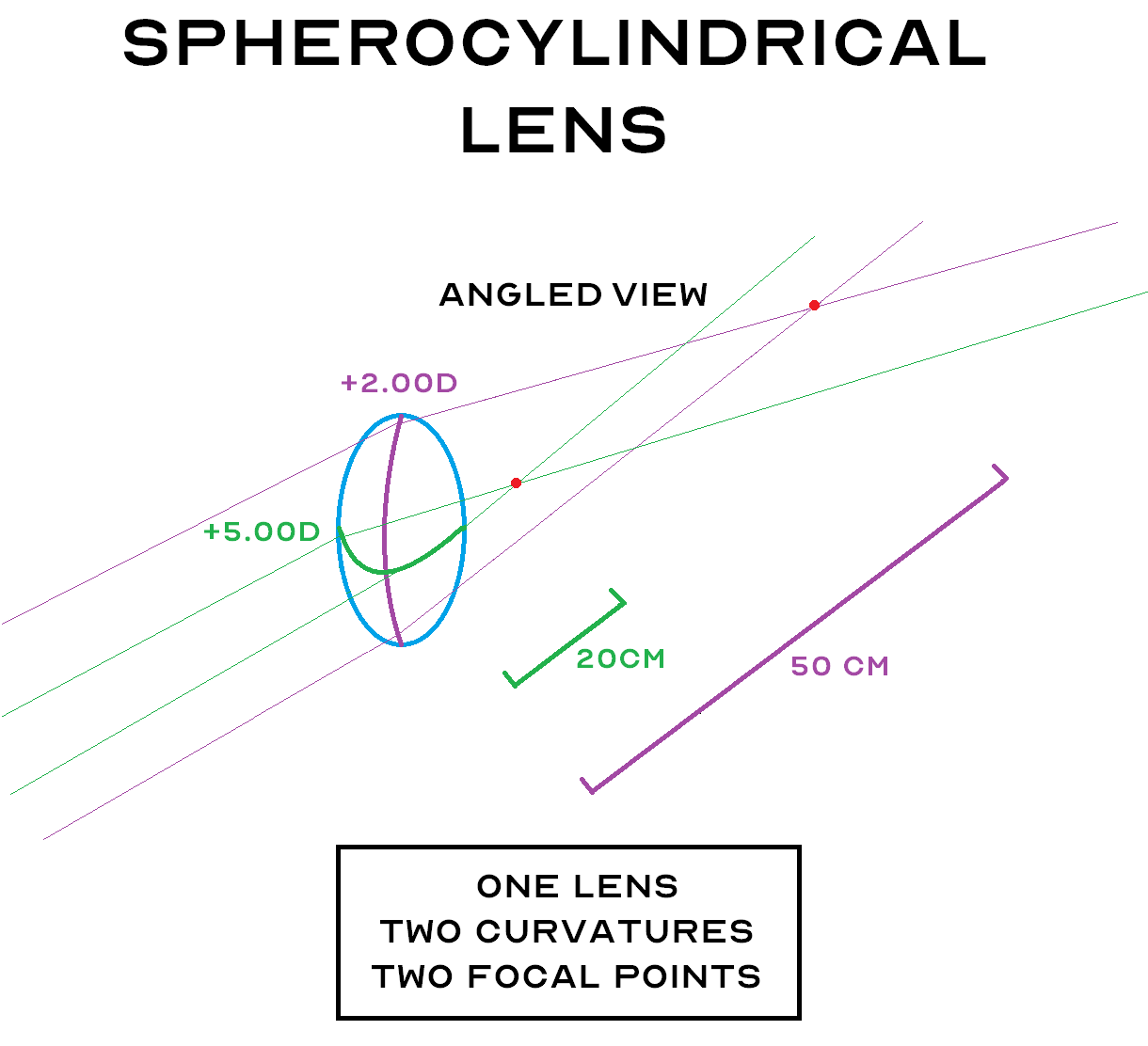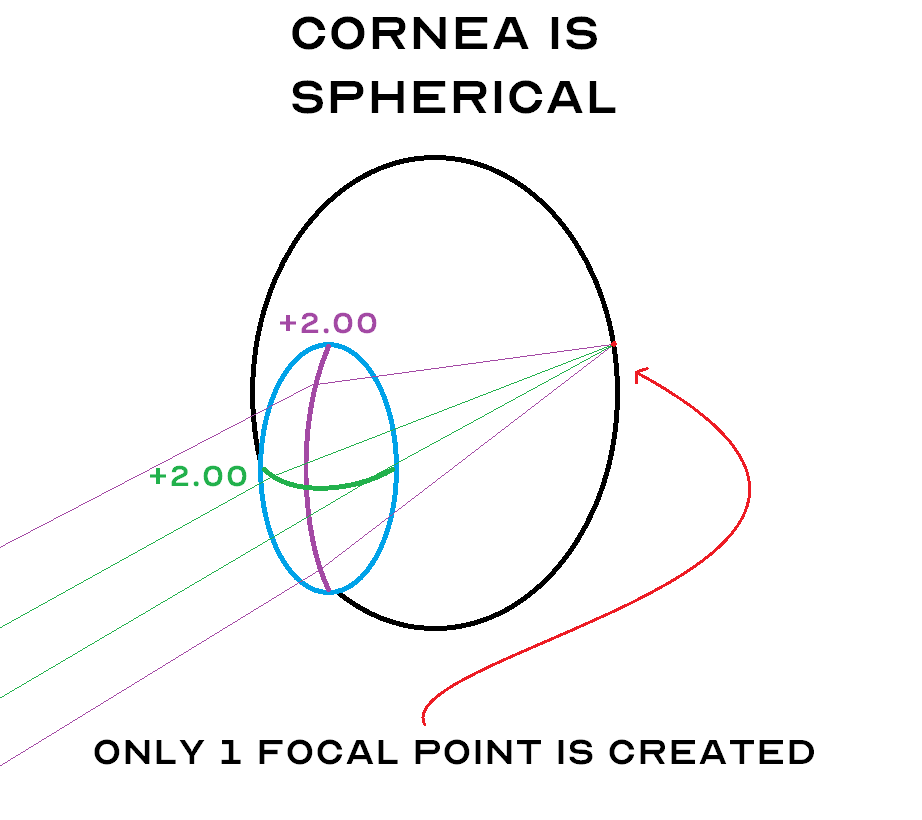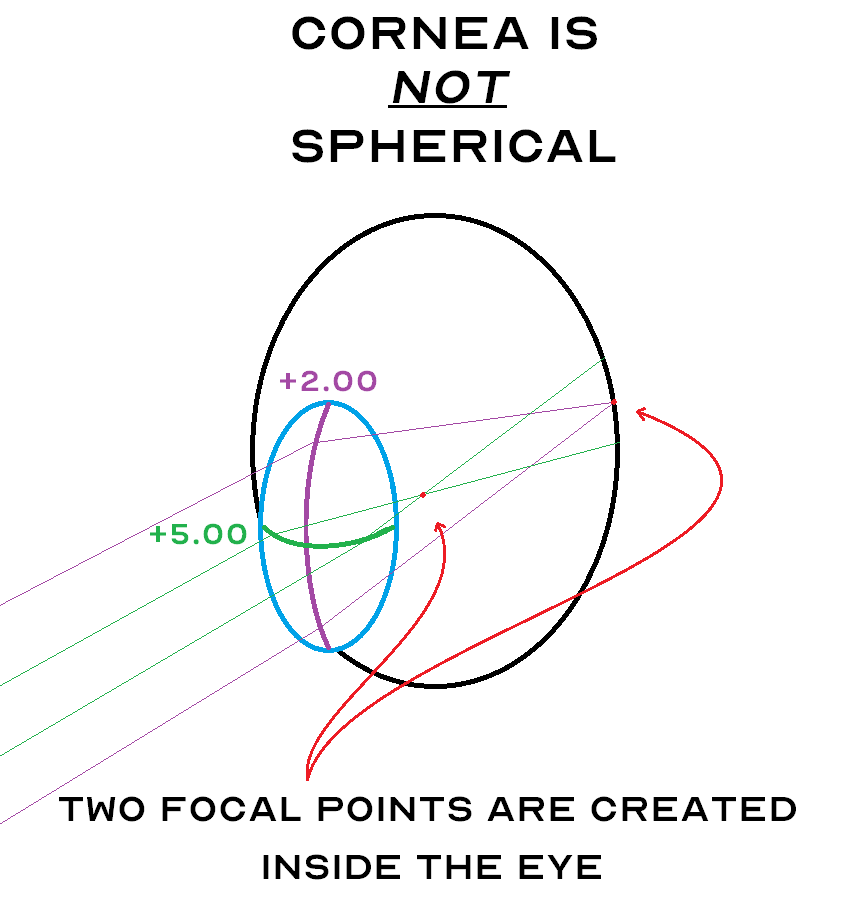After studying this lesson, you will understand a concept that eludes the vast majority of people – Astigmatism! But before you proceed, keep in mind that this is Lesson 8 of the course and it is highly advisable that you have read lessons 1-7 before tackling this one.

# Astigmatism

### What is Astigmatism?

Astigmatism is a refractive condition that results in the creation of 2 separate focal points.

Recall from Lesson 6 & Lesson 7 that the difference between emmetropia, myopia and hyperopia is where the focal point forms inside the eye.

• In emmetropia the focal point forms on the retina
• In myopia the focal point forms in front of the retina
• In hyperopia the focal point forms behind the retina

In all 3 of these conditions the eye produces only a single focal point. But what if there were 2 focal points…? Then that would be astigmatism!

### How Can There Be 2 Focal Points?

Brilliant question!

Everything that you have learned in this course so far suggests that it is impossible for a lens to produce two focal points.Question: So how can one lens produce two different focal points?

Answer: It must have two different curvatures!

Up until now we have always assumed that the lenses and the cornea in our examples have only one curvature, but this is not always true. A lens can have two different curvatures.

# Spherical Vs Spherocylindrical Lenses

A lens that has two different curvatures is called a spherocylindrical lens. Before we delve into spherocylindrical lenses, let’s make sure we understand simple spherical lenses.

## Spherical Lens

A spherical lens is simply a lens that has the same curvature all around. This is the type of lens we’ve been dealing with in all of our lessons so far.

In the following diagram, you will see a +2.00D spherical lens from two different perspectives. The top is the side view you’re familiar with, but the bottom is an approximately 45° angle view. That’s why it appears oval in nature, but keep in mind that it is the same lens.There’s a lot going on in this diagram so let’s examine it closely.

Notice that:

• Both top and bottom lenses have the same power; +2.00D
• Both top and bottom lenses have the same focal distance; 50 cm
• In a side view (top) only one curvature can be seen; the vertical curvature
• In a 45° angle view, two curvatures can be seen; the vertical curvature (purple) and the horizontal curvature (green)

In this diagram, we see a lens with a vertical and horizontal curvature that are the same. That’s what makes this a spherical lens.

#### A spherical lens has the same vertical and horizontal curvature

Note: Just because the horizontal view (top) shows a lens that appears to only have a vertical curvature, it does not mean that it has no horizontal curvature. The horizontal curvature is just not visible from that perspective. When drawn this way, the horizontal curvature is simply assumed to be the same as the vertical curvature.

Now let’s see what happens when the vertical and horizontal curvatures are not the same.

## Spherocylindrical Lens

A spherocylindrical lens is different from a spherical lens in that:

• They have two different curvatures
• The different curvatures are always 90° apart
• Each curvatures creates a separate focal point

The following diagram illustrates a spherocylindrical lens.Notice that this lens has two different curvatures instead of just one. The vertical curvature (purple) corresponds to a +2.00D lens power and the horizontal curvature (green) corresponds to a +5.00D lens power.

When light passes through this spherocylindrical lens, each curve will receive exactly half of the light rays.

• The rays that pass through the +2.00D curve get focused at 50cm from the lens
• The rays that pass through the +5.00D curve get focused at 20cm from the lens

# The Cornea As A Lens

Remember that the cornea acts as a lens for the eye – and yes – it can either be spherical or spherocylindrical lens!

• If the cornea is spherical – the eye has no astigmatism
• If the cornea is spherocylindrical – the eye has astigmatism

First, let’s look at a spherical cornea. Remember, this means there is no astigmatism.The cornea is the blue circle. It appears oval because again, we are looking at a 45° angle. If we looked at it straight on it would be a perfect circle.

This cornea has the same curvature in both directions. This makes it a spherical cornea. That’s why it only produces one focal point inside the eye.

Now let’s look at a shperocylindrical cornea.Because this cornea has two different curvatures, it creates two separate focal points inside the eye. That is astigmatism.

# What Does Astigmatism Mean For You?

If you have astigmatism, it means that the glasses or contact lenses that you wear will have to correct (with spherocylindrical lenses) for both curvatures of the cornea so that there is only one focal point inside your eye. Remember from Lesson 5 that for an eye to see clearly, there must only be only one focal point and it has to form on the retina. If there are two focal points inside the eye, the eye will not see clearly.

Glasses for astigmatism are no different than glasses that are not for astigmatism. On the other hand, contact lenses for astigmatism are specialized contact lenses that are more expensive and may be more difficult to wear successfully.

# Summary

Astigmatism is not an easy concept to grasp. This is a lesson I highly recommend reading a few times as it is quite complex. If you still have questions I would be happy to answer them for you, just let me know in the comments below!

If you’re comfortable with this lesson, congratulations! You now possess knowledge that very few people possess, so use it wisely! You’ll certainly find that it will come in handy if you work in an eye doctor’s office or an optical store.

In the next lesson we’re going to learn about a new part of the eye and how it affects vision. It’s called the crystalline lens. See you there!